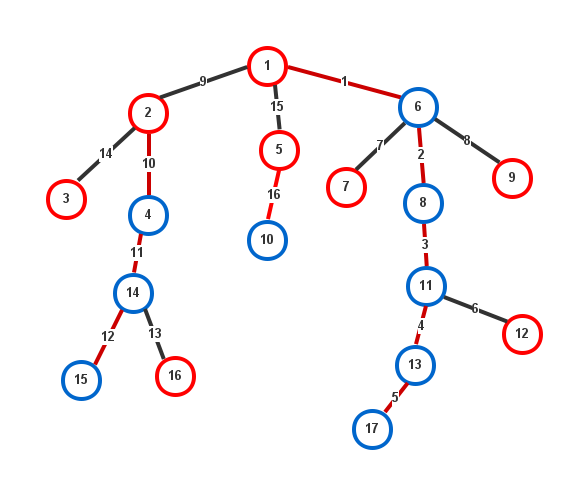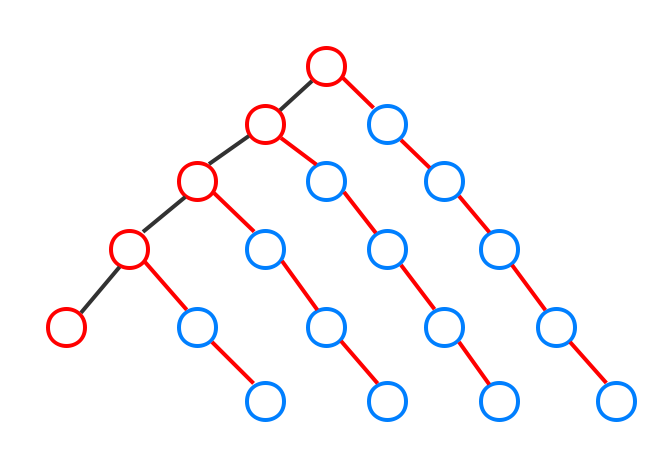# 树链剖分

## 用途

• 查询$u$$v的路径上边权之和 • 查询u$$v$的路径上边权最大值
• 修改$u$$v的路径上每条边的边权。 这些操作利用树链剖分，都可以在O(\log n)的时间内解决。 ## 无根树与有根树 一般来说，无根树是很不好处理的，因此将其转为有根树就会好处理些。 我们只要选定一个节点为根，然后进行DFS即可转化为有根树。 转换的时候，我们需要额外记录以下信息： • x.\text{father}: x的父亲节点。 • x.\text{children}: x的所有孩子。 • x.\text{size}: 以x为根的这一棵子树的大小。 • x.\text{depth}: x在树中的深度，即x到树根的距离+1这是一棵以1为根的树。为了解释更加清楚，这里举几个例子： • 10.\text{father} = 5,\; 1.\text{father} = nil • 1.\text{children} = \{2, 5, 6\},\;7.\text{children} = \varnothing • 1.\text{size} = 17,\;6.\text{size} = 8,\;3.\text{size} = 1 • 1.\text{depth} = 1,\;10.\text{depth} = 3,\;14.\text{depth} = 4 为了进行树链剖分，我们在立树的过程中需要计算一个重儿子，记作x.\text{next}$$ x.\text{next} = u: u \in x.\text{children},\;u.\text{size}\text{最大} $$即所有儿子中大小最大的一个，就记为重儿子，其它的儿子就是轻儿子。同时称与重儿子相连的边为重边，与轻儿子相连的边为轻边 下面的伪代码是建立有根树的过程(假设我们输入的是无根树G，这是一张无向图，其中与u相连的顶点集合为G[u].\text{neighbors})：   1 2 3 4 5 6 7 8 9 10 11 12 13 14 15 16 17 18 19 20 21 22 23 24 function MAKE-ROOT(G, u): // 以u为树根 u.visited = true // u已经访问过了 u.father = nil // u没有父亲 u.depth = 1 REAL-MAKE-ROOT(G, u) function REAL-MAKE-ROOT(G, u): // DFS过程 u.size = 1 u.next = nil foreach v in G[u].neighbors: if not v.visited: // 如果还未访问 v.visited = true u.children += v // 添加儿子节点 v.father = u v.depth = u.depth + 1 REAL-MAKE-ROOT(G, v) // 递归向下 u.size += v.size // 更新子树大小 if u.next = nil || v.size > u.next.size: // 更新重儿子 u.next = v  ## 剖分 在介绍树链剖分的算法之前，我们先来看看剖分后的树是个什么样子：剖分后的树信息量一下子大了很多。我将会逐条解释上面都画了些什么。 • 树链(重链): 所有红色的边所连成的一条链都是剖分后的结果。某些树链没有边，就只有一个节点，这个节点是红色的。在上面，1-6-8-11-13-17是剖分出来的最长的树链，而3独自一个节点形成了树链。 • 树链起点: 红色的节点是每一条树链的起点。 • 重儿子: 蓝色的节点是重儿子。 • 编号: 每一条边上的数字为剖分后的编号。 可以看出，树中的每一个节点都会在一条树链中。因此我们需要对树链也进行编号。我们以树链顶端的节点作为树链的编号，并记为x.\text{top}。例如，8.\text{top} = 1,\;16.\text{top} = 16 如果两个节点u$$v$在同一树链中，当且仅当$u.\text{top} = v.\text{top}$

  1 2 3 4 5 6 7 8 9 10 11 12 13 14 15 16 17 18 19 20 21 22 23 count = 0 // 已编号的数量 function TREE-SPLIT(x): // 剖分子树u x.id = 0 // 根节点没有父亲 x.top = x // 根节点是第一条链 REAL-TREE-SPLIT(x) function REAL-TREE-SPLIT(x): // DFS过程 if x.next != nil: // 如果有重儿子 count += 1 x.next.id = count x.next.top = x.top REAL-TREE-SPLIT(x.next) // 优先重儿子 foreach v in x.children: // 对于轻儿子则新创一条链 if v != x.next: // 检查是不是重儿子 count += 1 v.id = count v.top = v REAL-TREE-SPLIT(v) 

## 基础操作

• 查询$u$$v的路径上边权之和 • 查询u$$v$的路径上边权最大值
• 修改$u$$v的路径上每条边的边权。 下面将介绍如何进行路径边权之和的查询修改路径边权这两个操作。其它的操作可以在此思想上扩展。 ### 查询 假如我们要查询u$$v$的路径上的权值之和，那么就会有两种情况：

• $u$$v同一条树链上。 • u$$v$不在同一条树链上。

• 计算$[u.\text{top}.\text{id},u.\text{id}]$的值并累加
• $u = u.\text{top}.\text{father}$
• 如果$u.\text{top} = v.\text{top}$，则转化为第一种情况。否则跳转第一步。

  1 2 3 4 5 6 7 8 9 10 11 12 13 14 15 16 17 18 function QUERY-SUM(u, v): sum = 0 // 如果不在同一条树链 while u.top != v.top: if u.top.depth < v.top.depth: SWAP(u, v) // 交换u和v sum += QUERY(u.top.id, u.id) // 利用线段树等数据结构来求和 u = u.top.father // 走轻边进入上面的树链 if u == v: // 如果处在相同位置 return sum if u.depth > v.depth: // 使u成为深度较小的节点 SWAP(u, v) return QUERY(u.next.id, v.id) + sum## 时间复杂度## 树链剖分计算LCA

LCA即最近公共祖先。计算LCA的算法可以说是各种各样，有暴力的爬山法，炸空间的Tarjan算法，开挂的倍增法，莫名高大上的ST跳表的搞法，还有不知所云的转成RMQ问题......在与树相关的操作中，经常需要一些求LCA的操作。其中最常用的就是倍增法，它能在$O(\log n)$的时间内求出LCA，并且其空间复杂度为$\Theta(n \log n)$

## 树链剖分与DFS序

【NOI2015】软件包管理器

1. 实际上，按照子树大小来剖分不一定能剖分出最长的树链，但这并不影响最终的操作的效率。如果要剖分出的链真正最长，应当选择秩最大的儿子作为重儿子。